# Sqrt

Output the square root of the input (input >= 0 required)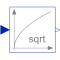# Information

This information is part of the Modelica Standard Library maintained by the Modelica Association.

This blocks computes the output y as square root of the input u:

```    y = sqrt( u );
```

The input shall be zero or positive. Otherwise an error occurs.

# Connectors (2)

u y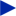Type: RealInput Description: Connector of Real input signal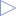Type: RealOutput Description: Connector of Real output signal

# Used in Components (2)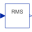RootMeanSquare Modelica.Blocks.Math Calculate root mean square over period 1/f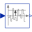StandardDeviation Modelica.Blocks.Math Calculates the empirical standard deviation of its input signal